Request a call back

# ICSE Class 10 Mathematics Previous Year Question Paper 2012

ICSE Class 10 Maths is challenging yet one of the high-scoring subjects. ICSE Class 10 is a critical phase in a student's school life. The students need to focus on the vital subjects because the scores obtained by the students in ICSE Class 10  board examination are the basis for their future admissions in leading universities and institutions. As there is a vast syllabus, the students require expert-curated study resources for concept-based learning Class 10 ICSE Mathematics. The complete syllabus as per the ICSE curriculum is available at ICSE Class 10 Maths Syllabus. Students should kick out the fear concerning the Class 10th ICSE Mathematics syllabus from their minds by having a sound conceptual understanding of the subject. ICSE 10 Maths learning solutions can help students learn complicated topics like Quadratic Equations, Trigonometry, Mensuration, and Arithmetic & Geometric Progressions with ease. Moreover, students can escalate their scores with our enhanced learning modules for ICSE Class 10 Maths.

TopperLearning focuses on specific solutions and study material that are aligned with the guidelines by the  ICSE Board guidelines. Notably, our well-organized. ICSE Class 10 Plans are accessible online, where you can choose from different plans as per your needs. So to enroll and get free access to our comprehensive learning resources anytime, anywhere. In addition, we provide you with integrated ICSE Class 10 Exam preparation study material that will lead you to gain outstanding results.

TopperLearning offers specialized study resources designed by experts to assist the students' in effective learning in qualifying their examinations with impressive scores. We provide online access to all our Class 10 ICSE study materials, including practice papers, Selina solutions for ICSE Class 10, video lessons, and much more.

Question 1

If (x + 2) is a factor of the polynomial x3 - kx2 - 5x + 6 then the value of k is:                             

1. 1
2. 2
3. -2

Question 2

The solution set of the inequation x - 3 ≥ - 5, x ∊ R is:                                                                       

1. {x : x > - 2, x ∊ R}
2. {x : x ≤ -2, x ∊ R}
3. {x : x  ≥ -2, x ∊ R}
4. {-2, -1, 0, 1, 2}

Question 3

The product AB of two matrices A and B is possible if:                                   

1. A and B have the same number of rows.
2. The number of columns of A is equal to the number of rows of B.
3. The number of rows of A is equal to the number of columns of B.
4. A and B have the same number of columns.

Question 4

If 70, 75, 80, 85 are the first four terms of an Arithmetic Progression. Then the 10th term is:       

1. 35
2. 25
3. 115
4. 105

Question 5

The selling price of a shirt excluding GST is Rs. 800. If the rate of GST is 12% then the total price of the shirt is:        

1. Rs. 704
2. Rs. 96
3. Rs. 896
4. Rs. 848

Question 6

Which of the following quadratic equations has 2 end 3 as its roots?                

1. x2 - 5x + 6 = 0
2. x2 + 5x + 6 = 0
3. x2 - 5x - 6 = 0
4. x2 + 5x -6 = 0

Question 7

If x, 5.4, 5, 9 are in proportion then x is:               

1. 9.72
2. 25
3. 25/3

Question 8

Mohit opened a Recurring deposit account in a bank for 2 years. He deposits Rs. 1000 every month and receives Rs. 25500 on maturity. The interest he earned in 2 years is:    

1. Rs. 13500
2. Rs. 3000
3. Rs. 24000
4. Rs. 1500

Question 9

In the given figure AB = 24 cm, AC = 18 cm, DE = 12cm, DF = 9 cm and ∠BAC = ∠EDF. Then ΔABC ~ ΔDEF by the condition: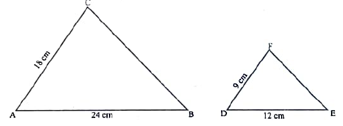1. AAA
2. SAS
3. SSS
4. AAS

Question 101.2.3.4.Question 11

The polynomial x3 - 2x2 + ax + 12 when divided by (x + 1) leaves a remainder 20, then 'a' is equal to:

1. - 31
2. 11
3. - 11

Question 12

In an Arithmetic Progression (A.P.) if, first term is 5, common difference is - 3 and then nth term is - 7, then n is equal to:

1. 17
2. - 13
3. 7

Question 13

In the given figure PQ is parallel to TR, then by using condition of similarity: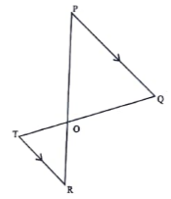1.2.3.4.Question 14

If a, b, c, and d are proportional thenis equal to:                           

1.2.3.4.Question 15

The first four terms of an Arithmetic Progression (A. P.), whose first term is 4 and common difference is -6, are:            

1. 4, -10, -16, -22
2. 4, 10, 16, 22
3. 4, -2, -8, -14
4. 4, 2, 8, 14

Question 16

One of the roots of the quadratic equation x2 – 8x + 5 = 0 is 7.3166. The root of the equation correct to 4 significant figures is:       

1. 7.3166
2. 7.317
3. 7.316
4. 7.32

Question 17

(x + 2) and (x + 3) are two factors of the polynomial x3 + 6x2 + 11x + 6. If this polynomial is completely factorised the result is:             

1. (x – 2)(x + 3)(x + 1)
2. (x + 2)(x – 3)(x – 1)
3. (x + 2)(x + 3)(x – 1)
4. (x + 2)(x + 3)(x + 1)

Question 18

The sum of the first 20 terms of the Arithmetic Progression 2, 4, 6, 8,....is :                                                      

1. 400
2. 840
3. 420
4. 800

Question 19

The solution set on the number line of the linear inequation:                                                

2y – 6 < y + 2 ≤ 2y, y ∈ N is

1.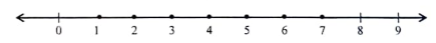2.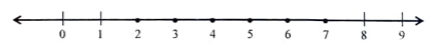3.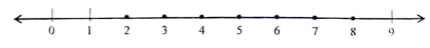4.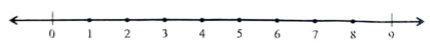Question 20

If x, y, z are in continued proportion then (y2 + z2): (x2 + y2) is equal to:                             

1. z : x
2. x : z
3. zx
4. (y + z) : (x + y)

Question 21

The marked price of an article is Rs. 5,000. The shopkeeper gives a discount of 10%. If the rate of GST is 12%, then the amount paid by the customer including GST is:    
1. Rs. 5040
2. Rs. 6100
3. Rs. 6272
4. Rs. 6160

Question 22

If A =then 5A – BC is equal to:                        

1.2.3.4.Question 23

In the given figure ABCD is a trapezium in which DC is parallel to AB.
AB = 16 cm and DC = 8 cm. OD = 5 cm, OB = (y + 3) cm, OA = 11 cm and OC = (x – 1) cm.
Using the given information answer the following questions.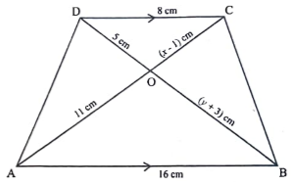i. From the given figure name the pair of similar triangles:                   
1. ∆OAB, ∆OBC
2. ∆COD, ∆AOB
4. ∆COD, ∆COB

ii. The corresponding proportional sides with respect to the pair of similar triangles obtained in (i):        
1.2.3.4.iii. The ratio of the sides of the pair of similar triangles is:                    
1. 1 : 3
2. 1 : 2
3. 2 : 3
4. 3 : 1

iv. Using the ratio of sides of the pair of similar triangles the values of x and y are respectively:                   
1. x = 4.6, y = 7
2. x = 7, y = 7
3. x = 6.5, y = 7
4. x = 6.5, y = 2

Question 24

Two cars X and Y use 1 litre of diesel to travel x km and (x + 3) km respectively. If both the cars covered a distance of 72 km, then:

i. The number of litres of diesel used by car X is :         
1.2.3.4.ii. The number of litres of diesel used by car Y is:                                
1.2.3.4.iii. If car X used 4 litres of diesel more than car Y in the journey, then:              
1.2.3.4.iv. The amount of diesel used by the car X is:                 
1. 6 litres
2. 12 litres
3. 18 litres
4. 24 litres

Question 25

Joseph has a recurring deposit account in a bank for two years at the rate of 8% per annum simple interest

i. If at the time of maturity Joseph receives Rs. 2000 as interest then the mostly instalment is:        
1. Rs. 1200
2. Rs. 600
3. Rs. 1000
4. Rs. 1600

ii. The total amount deposited in the bank:                         
1. Rs. 25000
2. Rs. 24000
3. Rs. 26000
4. Rs. 23000

iii. The amount Joseph receives on maturity is:               
1. Rs. 27000
2. Rs. 25000
3. Rs. 26000
4. Rs. 28000

iv. If the monthly instalment is Rs. 100 and the rate of interest is 8%, in how many months Joseph will receive Rs. 52 as interest?    
1. 18
2. 30
3. 12
4. 6

## Why to choose our study materials for ICSE Class 10 Mathematics?

• Designed in simple and comprehensive language
• Contain 285 video lessons, 37 revision notes, 3709+ questions in bank, set of 6 sample papers and 9 past year papers
• Prepared by subject experts
• Revised from time to time
• Useful for doing quick revision